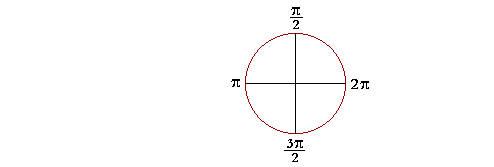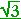13

Coterminal angles

The multiples of π

IN THE RADIAN SYSTEM of angular measurement, the measure of one revolution is 2π.

(In the next Topic, Arc Length, we will see the actual definition of radian measure.)Half a circle, then, is π.  And, most important, each right angle is half

 of π: π2 .
 Three right angles will be  3· π2 = 3π 2 .
 Five right angles will be 5π 2 .  And so on.

The following radian measures come up frequently, and the student should know their degree equivalents:(Topic 4 and Topic 5.)

 π4 is half of π2 , a right angle, and so it is equal to 45°.   (Skill in
 Equivalently, π4 is of one quarter of π, or half of half of 180°.
 π3 is a third of π, and so is equal to 180° ÷ 3 = 60°.
 π6 is a sixth of π, and so is equal to 180° ÷ 6 = 30°.5π 4 = 5· π4 = 5· 45° = 225°.
 2π 3 is a third of 2π.   A third of a revolution = 360° ÷ 3 = 120°.

Problem 1 .   Convert each of these radian measures into degrees.

Problem 1.    The student should know these.

To see the answer, pass your mouse over the colored area.
To cover the answer again, click "Refresh" ("Reload").

 a)   π  180° b) π2 90° c) π3 60° d) π6 30° e) π4 45°

Problem 2.   Convert each of these radian measures into degrees.

 a) π8 22½°. π8 is half of π4 .
 b) 2π 5 72°. 2π 5 is a fifth of 2π , which is a fifth of a 360°.
 c) 7π 4 = 7· π4 = 7· 45° = 315°
 d) 9π 2 = 9· π2 = 9· 90° = 810°
 e) 4π 3 = 4· π3 = 4· 60° = 240°
 f) 5π 6 = 5· π6 = 5· 30° = 150°
 g) 7π 9Problem 3.   Evaluate the following.  (See Topic 4 and Topic 5.)

 a)  cos π6 =2 b)  sin π6 = 12 c)  tan π4 = 1 d)  cot π3 = 1e)  csc π6 = 2 f)  sec π4 =Problem 4.   In terms of radians, what angle is the complement of an

 angle θ ? π2 − θ

Problem 5.   A function of any angle is equal to the cofunction of its complement. (Topic 3.)  Therefore, in terms of cofunctions:

 a)  sin θ = cos ( π2 − θ ) b)  cot θ = tan ( π2 − θ )
 c)  sec ( π2 − θ) = csc θ

360°  =  2π.

When we write 2π, we mean 2π radians, which is approximately 6.28 radians.  However, we normally omit the word radians.  As we will see in the next Topic, Arc length, the radian measure can be any real number.

Problem 6.   The student should begin by knowing these.

 0°  = 0 radians. 360° = 2π. 180° = π. 90° = π2 .
 45° = π4 . 60° = π3 . 30° = π6 .

Example 1.   Convert 120° into radians.

Solution.   We can go from what we know to what we do not know.  In the most important cases we can recognize the number of degrees as a multiple of 90°, or 45°, or 60°, or 30°; or as a part of 360°.

 Since 60° = π3 , then
 120° = 2· 60° = 2· π3 = 2π 3 .

Or, since 120° is a third of 360°, which is 2π, then

 120°  = 2π 3 .
 Example 2.   225° = 180° + 45°  =  π + π4 = 5π 4 .

Or,

 225° = 5· 45°  =  5· π4 = 5π 4 .

Problem 7.   Convert each of the following into radians.

 a)  270° = 3· 90° = 3π 2 b)  210° = 7· 30° = 7· π6 = 7π 6
 c)  300° = 5· 60° = 5· π3 = 5π 3 d)  135° = 3· 45° = 3· π4 = 3π 4
 e)  720° = 2· 360° = 2· 2π = 4π
 f)  450° = 5· 90° = 5· π2 = 5π 2
 g)  36°  = A tenth of 360° = 2π10 = π5
 h)  72°  = 2· 36° = 2π 5

72° is thus a fifth of a revolution.

 i)  40°  = A ninth of 360° = 2π 9
 j)  80°  = 2· 40° = 4π 9

As a last resort, proportionally,so thatExample 3.   Change 140° to radians.

 Solution. 140180 · π = 79 · π = 7π 9 ,

upon dividing both the numerator and denominator first by 10 and then by 2.  (Lesson 21 and Lesson 1 of Arithmetic.)

Coterminal angles

Angles are coterminal if they have the same terminal side.θ is coterminal with −φ.  They have the same terminal side.

Notice that

θ + φ  =  2π,

so that

θ  =  2πφ .  .  .  .  .  .  .  . (1)

Example 4.   Name in radians the non-negative angle that is coterminal

 with − 2π 5 , and is less than 2π.

Answer.  Let us call that angle θ.  Then according to line (1),

 θ  =  2π − 2π 5 = 10π − 2π        5 = 8π 5

Problem 8.   Name in radians the non-negative angle that is coterminal with each of the following, and is less than 2π.

 a)  − π6 θ  =  2π − π6 = 12π − π     6 = 11π  6
 b)  − 3π 4 θ = 2π − 3π 4 = 8π − 3π     4 = 5π 4
 c)  − 4π 3 θ  =  2π − 4π 3 = 6π − 4π     3 = 2π 3

Starting at 0, let us go around the circle a half-circle at a time.  We will then have the following sequence, which are the multiples of π:

0,  π,  2π,  3π,  4π, 5π, etc.The point to see is that the odd multiples of π --

π,  3π,  5π,  7π, etc.

-- are coterminal with π.  While the even multiples of π --

2π,  4π,  6π, etc.

-- are coterminal with 0.

If we go around in the negative direction,we can make a similar observation.

Problem 9.   Name in radians the non-negative angle that is coterminal with each of the following, and is less than 2π.

a)   -π   π       b)   -2π        c)   -3π   π       d)   -4π        e)   -5π   π

f)   3π   π        g)   4π         h)   5π   π        i)   6π         j)   7π   π

Next Topic:  Arc Length

Please make a donation to keep TheMathPage online.
Even \$1 will help.